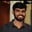Related Tags

c

# What is a one-dimensional array?Buchiredddypalli Koushik

## Overview

In this shot, we will read one array using the standard input (scanf) in C. The array’s size is also read from the user, and we will print the final array.

### What is an array?

An array is a group of elements or data. The elements are stored in the contiguous memory location.

In arrays, we can have only a single data type element. We cannot store different datatype elements in the arrays.

### Syntax

• int a;

In the syntax above, int is the data type, a is the array’s name, and 5 is the array’s size.

If we want our array size to be read by the user, we use the following syntax:

• int ar[n];

Here, int is the data type, ar is the array’s name, and n represents the array’s size, which the user reads when executing the code.

### Pseudocode

Here is the pseudocode for taking the array as an input and printing that array:

for(i=0;i<n;i++)

{

scanf("%d",&a[i]);

}

for(i=0;i<n;i++)

{

printf("%d",arr[i])

}
The pseudocode to input and print a one-dimensional array in C

### Example

Let's view a code example:

#include<stdio.h>
int main()
{
int n;
scanf("%d",&n);
int i, arr[n]; //creates array arr of size 5
for (i = 0; i < n; i++) // loop from i = 0 to i = 4
{
scanf("%d", &arr[i] );//replace _ by arr[i] to take input for ith element
}
printf("The Elements of the array are : ");
for (i = 0; i < n; i++)
{
printf("%d ", arr[i] );//replace _ to print the ith element.
}
return 0;
}

Enter the input below

Implementation of an array in C

### Explanation

• Line 4: We declare n, which represents the array’s size.
• Line 6: We declare the array.
• Line 7–10: We read the array’s input.
• Line 12–15: We print the array’s elements.

RELATED TAGS

c

CONTRIBUTORBuchiredddypalli Koushik
RELATED COURSES

View all Courses

Keep Exploring

Learn in-demand tech skills in half the time